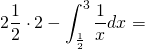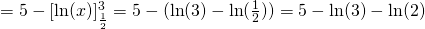# Solution assignment 02 Area and volume

### Assignment 2

Calculate the area which is enclosed by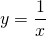, the lines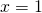,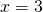and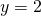.

### Solution

The area can be calculated as follows: calculate the area of the rectangle through the points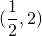and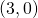and subtract the area of the area enclosed by the lines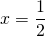and, the-axis and the graph of. This yields the following formula: